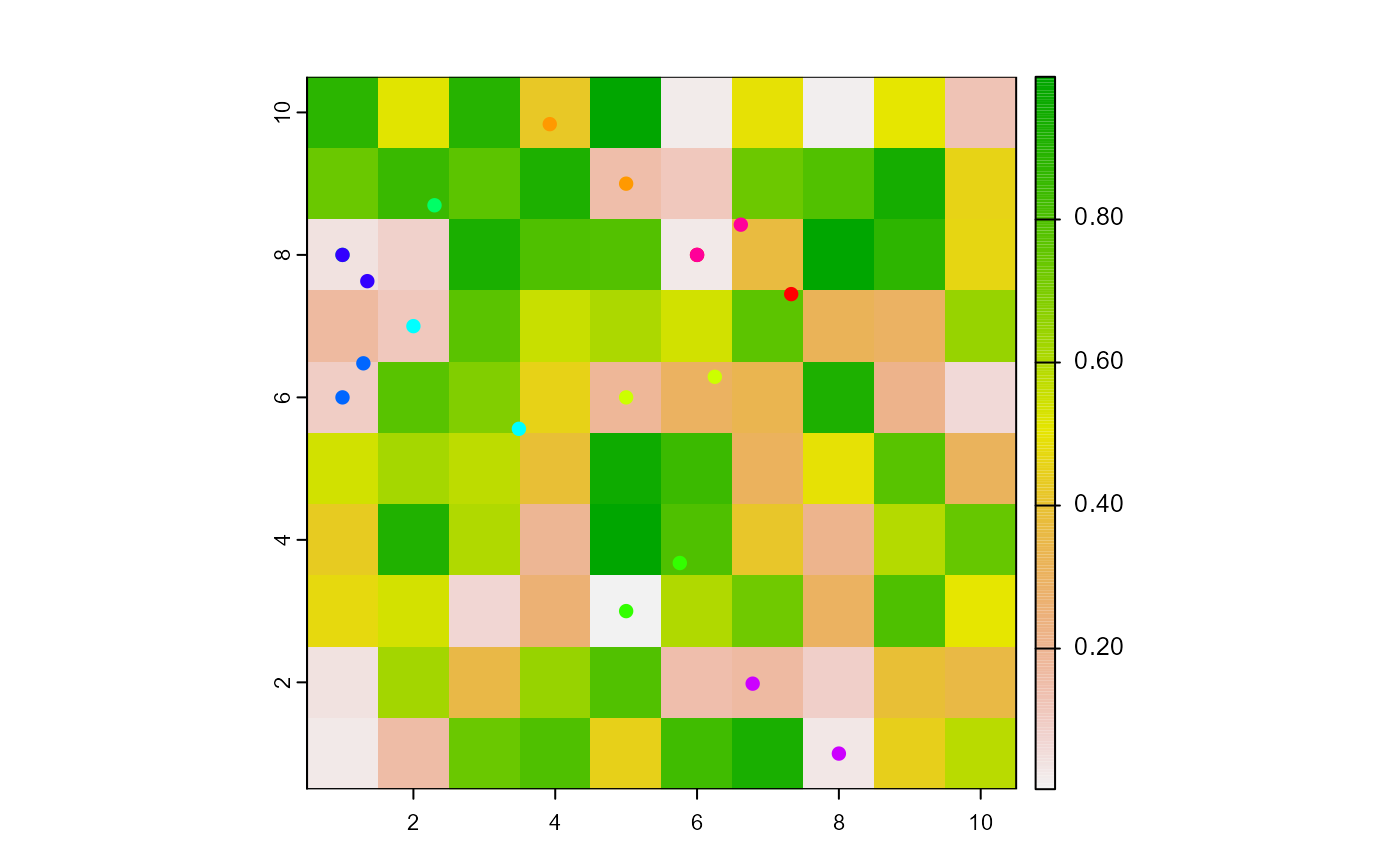Move the turtles to their neighboring patch with the lowest value.

downhill(world, pVar, turtles, nNeighbors, torus = FALSE)

# S4 method for worldMatrix,missing,agentMatrix,numeric
downhill(world, turtles, nNeighbors, torus)

# S4 method for worldArray,character,agentMatrix,numeric
downhill(world, pVar, turtles, nNeighbors, torus = FALSE)

## Arguments

world

WorldMatrix or worldArray object.

pVar

Character. If the world is a worldArray object, pVar is the name of the layer to use to define the patches values. pVar must not be provided if the world is a worldMatrix object.

turtles

AgentMatrix object representing the moving agents.

nNeighbors

Integer: 4 or 8. Represents the number of neighbor patches considered.

torus

Logical to determine if the world is wrapped. Default is torus = FALSE.

## Value

AgentMatrix representing the turtles with updated coordinates and updated data for their heading values and previous coordinates prevX

and prevY.

## Details

If no neighboring patch has a smaller value than the patch where the turtle is currently located on, the turtle stays on this patch. It still moves to the patch center if it was not already on it.

     If there are multiple neighboring patches with the same lowest value,

the turtle chooses one patch randomly.

If a turtle is located on a patch on the edge

of the world and torus = FALSE, it has fewer

neighboring patches as options to move than nNeighbors; if

torus = TRUE, the turtle can move on the other side of the world to

move downhill and its choice of neighboring patches is always equals to

nNeighbors.

Sarah Bauduin

## Examples

w1 <- createWorld(
minPxcor = 1, maxPxcor = 10, minPycor = 1, maxPycor = 10,
data = runif(100)
)
t1 <- createTurtles(n = 10, coords = randomXYcor(w1, n = 10))
plot(w1)
points(t1, col = of(agents = t1, var = "color"), pch = 16)

if (requireNamespace("SpaDES.tools", quietly = TRUE)) {
t1 <- downhill(world = w1, turtles = t1, nNeighbors = 8)
points(t1, col = of(agents = t1, var = "color"), pch = 16)
}